# Points to Remember

#### 10. Frenkel defect is the combination of vacancy and interstitial defects. Cations leave their actual lattice sites and occupy the interstitial space in the solid. Density remains the same in Frenkel defect.

• AgBr is the compound which shows both Schottky defect as well as Frenkel
defect.

11. Non-stoichiometric defect:
(i) Metal excess defect due to anion vacancies.
(ii) Metal excess defect due to presence of extra cations.
(iii) Metal deficiency due to absence of cations.
12. F-Center : In metal excess defect, electrons are trapped in the anion vacancies which act as colour centres, e.g., NaCl gives yellow colour when heated in sodium vapour.

13. Doping is the process of increasing the conductivity of intrinsic semiconductors by adding an appropriate amount of suitable impurity in Si or Ge.
* n-type semiconductors : Silicon or Germanium (group 14) doped with electron rich impurity (group 15 element like P or As). Here, conductivity is due to the extra electrons or delocalized electrons.
* p-type semiconductors : Silicon or Germanium (group 14) doped with group 13 elements like B or Al. Here, conductivity is due to positively charged electron holes.
* 13-15 group compounds, e.g., InSb, AlP, GaAs.
* 12-16 group compounds, e.g., ZnS, CdS, CdSe, HgTe.
* These compounds have average valence of four and are used in semiconductor devices.

14. Magnetic Properties
* Ferromagnetic substances : A few substances like iron, cobalt, nickel and CrO2 etc. are attracted very strongly by a magnetic eld. Such substances are called ferromagnetic substances.
All molecular domains are arranged permanently in the same direction under influence of magnetic field.* Antiferromagnetism : Substances like MnO showing antiferromagnetism have domain structure similar to ferromagnetism substances, but their domains are oppositely oriented and cancel out each others magnetic moment and so cannot be attracted towards magnet.
* Ferrimagnetism : When the magnetic moments of the domains in the substances are aligned in parallel and antiparallel directions in unequal number. These are weakly attracted by magnetic eld as compared to ferromagnetic
substances. For example, Fe3O4, MgF2O4 etc.
* Paramagnetic substances are weakly attracted by a magnetic eld. Examples are O2, Cu2+, Fe3+, Cr3+ which are paramagnetic due to the presence of unpaired one or more electrons. They lose their magnetism in the absence of magnetic field.
* Diamagnetic substances are weakly repelled by a magnetic eld. Examples are H2O, NaCl, C6H6 because they have all the electrons paired.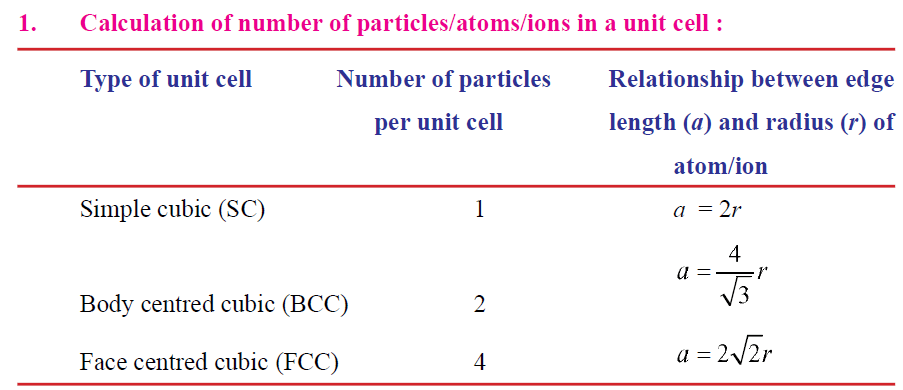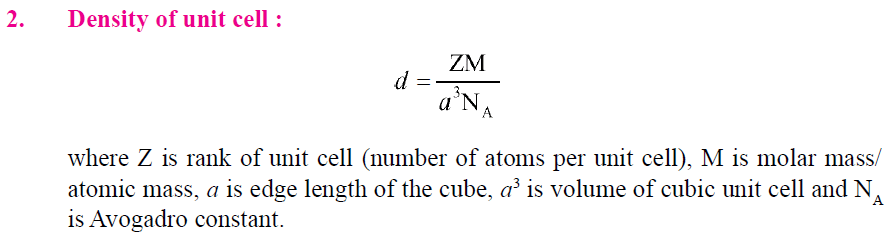VERY SHORT ANSWER TYPE QUESTIONS (1 Mark)
Q. 1. What do you mean by paramagnetic substance ?
Ans. Weakly attracted by magnetic eld and these substances are made of atoms or ions with unpaired electrons.
Q. 2. Which substance exhibit both Schottky and Frenkel defects ?
Ans. AgBr.
Q. 3. Name a salt which is added to AgCl so as to produce cationic vacancies.
Ans. CdCl2
Q. 4. Why Frenkel defects not found in pure alkali metal halides ?
Ans. Due to larger size of alkali metal ion, these cannot shift in interstitial space.
Q. 5. What is he use of amorphous silica ?
Ans. Used in photovoltanic cell.
Q. 6. Analysis shows that a metal oxide has the empirical formula M0.98O1.00.
Calculate the percentage of M2+ and M3+ ions in the crystal.
Ans. Let the M2+ ion in the crystal be x and M3+ = 0.98  x
Since, total charge on the compound must be zero,
So, 2x + 3(0.98  x)  z = 0
Or x = 0.94
% of M2+ =
% of M3+ = 100  97.9 = 2.1%
Q. 7. What is the co-ordination number of cation and anion in Caesium chloride
(bcc arrangement) ?
Ans. 8 and 8.
Q. 8. What is F-centre ?
Ans. It is the anion vacancy occupied by free electron in metal excess defect.
Q. 9. What makes alkali metal halides sometimes coloured, which are otherwise  colourless ?
Ans. Due to presence of F-centre.
Q.10. How does silica differ from quartz ?
Ans. Silica is amorphous form, while quartz is crystalline form of SiO2.
Q.11. Which point defect lowers the density of a crystal ?
Ans. Schottky defect/Vacancy defect.
Q.12.Why glass is called super cooled liquids ?
Ans. Hint : Its molecules move under gravity.
Q.13. Some of the very old glass objects appear slightly milky instead of being  transparent. Why ?
Ans. Re-allignment of molecules takes place due to movement because of constant
heating and cooling.
Q.14.What is anisotropy ?
Ans. Physical properties show different values when measured along different axis in
crystalline solids.
Q.15.What is the coordination number of atoms in : (a) fcc structure (b) bcc structure ?
Ans. (a) 12 (b) 8
Q.16. How many lattice points are there in one unit cell of :
(a) fcc
(b) bcc
(c) simple cubic arrangement ?
Ans. (a) 14 (b) 9 (c) 8
Q.17.What are the co-ordination numbers of octahedral voids and tetrahedral  voids ?
Ans. 6 and 4 respectively.
Q.18.Why common salt is sometimes yellow instead of being pure white ?
Ans. Due to the presence of electrons in some lattice sites in place of anions these sites
act as F-centres. These electrons when excited impart colour to the crystal.
Q.19.A compound is formed by two elements X and Y. The element Y forms ccp  arrangement and atoms of X occupy octahedral voids. What is the formula  of the compound ?
Ans. No. of Y (ccp) = 4
No. of X (octahedral void) = 4
X : Y = 4 : 4
XY

Q.20. Dene F-centres and how it is formed in lattice of crystal ?
Q.21. What type of stoichiometric defect is shown by
(a) ZnS
(b) AgBr
Q.22. What are the differences between Frenkel and Schottky defect ?
Q.23. Dene the following terms with suitable examples :
(a) Ferromagnetism
(b) Paramagnetism
(c) Ferrimagnetism
(d) 12-16 and 13-15 group compounds
Q.24. In terms of band theory, what is the difference :
(a) between conductor and an insulator ?
(b) between a conductor and a semi-conductor ?
Q.25. Explain how electrical neutrality is maintained in compounds showing
Frenkel and Schottky defect.
Ans. In compound showing Frenkel defect, ions just get displaced within the lattice,
while in compounds showing Schottky defect, equal number of anions and cations
are removed from the lattice. Thus, electrical neutrality is maintained in both
cases.
Q.26.Calculate the number of atoms in a cubic unit cell having one atom on each
corner and two atoms on each body diagonal.
Ans. 8 corner × 1/8 atom per unit cell = 1 atom
There are four body diagonals in a cubic unit cell and each has two body centre
atoms.
So, 4 × 2 = 8 atoms therefore, total number of atoms per unit cell = 1 + 8 = 9.
Q.27. Gold crystallizes in an FCC unit cell. What is the edge length of unit cell (r =
0.144 mm) ?
Ans. r = 0.144 nm

= 2 × 1.414 × 0.144 nm
= 0.407 nm
| 7
Q.28. Classify each of the following as either a p-type or n-type semi-conductor :
(a) Ge doped with In
(b) B doped with Si
Ans. Hint : (a) Ge is group 14 element and In is group 13 element. Therefore, an
electron deficit hole is created. Thus semi-conductor is p-type.
(b) Since B is group 13 element and Si is group 14 element, there will be a free
electron, thus, it is n-type semi-conductor.
Q.29. In terms of band theory, what is the difference between a conductor, an
insulator and a semi-conductor ?
Ans. The energy gap between the valence band and conduction band in an insulator is
very large while in a conductor, the energy gap is very small or there is overlapping
between valence band and conduction band.
Q.30. CaCl2 will introduce Schottky defect if added to AgCl crystal. Explain.
Ans. Two Ag+ ions will be replaced by one Ca2+ ion to maintain electrical neutrality.
Thus, a hole is created at the lattice site for every Ca2+ ion introduced.
Q.31. The electrical conductivity of a metal decreases with rise in temperature
while that of a semi-conductor increases. Explain.
Ans. In metals with increase of temperature, the kernels start vibrating at faster rate
and thus offer resistance to the flow of electrons. Hence, conductivity decreases.
In case of semi-conductors, with increase of temperature, more electrons can shift
from valence band to conduction band. Hence conductivity increases.
Q.32. What type of substances would make better permanent magnets 
ferromagnetic or ferromagnetic ? Why ?
Ans. Ferromagnetic substances make better permanent magnets. This is because the
metal ions of a ferromagnetic substance are grouped into small regions called
domains. Each domain acts as tiny magnet and get oriented in the direction of
magnetic eld in which it is placed. This persists even in the absence of magnetic
eld.
Q.33. In a crystalline solid, the atoms A and B are arranged as follows :
(a) Atoms A are arranged in ccp array.
(b) Atoms B occupy all the octahedral voids and half of the tetrahedral
voids.
What is the formula of the compound ?
Ans. No. of A (ccp) = 4
No. of B = octahedral voids + ( Tetrahedral / 2 )

= 4 + (8 /2)

= 8

A : B
4 : 8
1 : 2
therefore, formula of the compound is AB2Q.35. How many lattice points are there in one unit cell of the following lattices :
(a) FCC (b) BCC (c) SCC
Q.36. A cubic solid is made of two elements X and Y. Atom Y are at the corners of
the cube and X at the body centres. What is the formula of the compound ?
Ans. [Hint : XY]
Q.37. Silver forms ccp lattice and X-ray studies of its crystal show that the edge
length of its unit cell is 408.6 pm. Calculate the density of silver (Atomic wt.
= 107.9u).
Ans. [Hint : PQ, 8 and 9]
Q.38.A cubic solid is made up of two elements P and Q. Atoms of the Q are present at the corners of the cube and atoms of P at the body centre. What is the formula of the compound ? What are the co-ordination number of P and Q ?
Ans. [Hint : PQ, 8 ]
Q.39.What happens when :
(a) CsCl crystal is heated. (Hint : Changes to NaCl type crystal)
(b) Pressure is applied on NaCl crystal. (Hint : Changes to CsCl type crystal)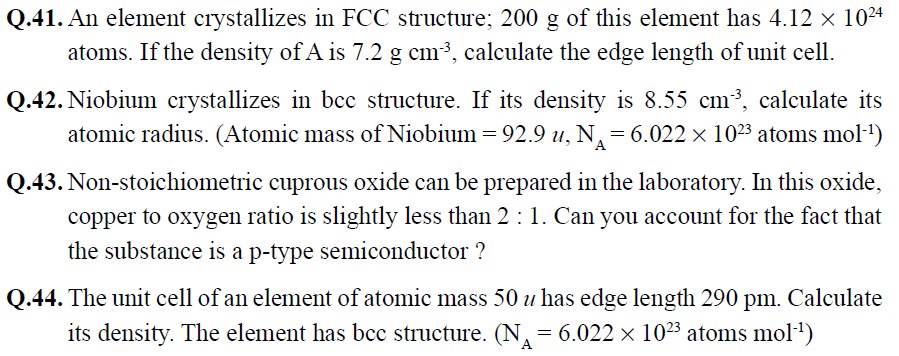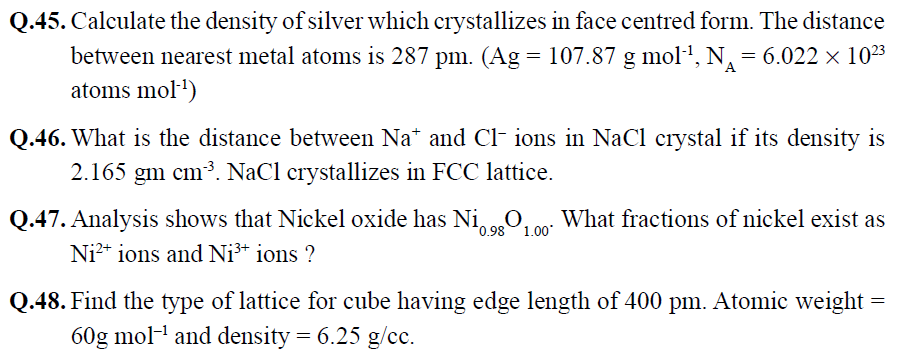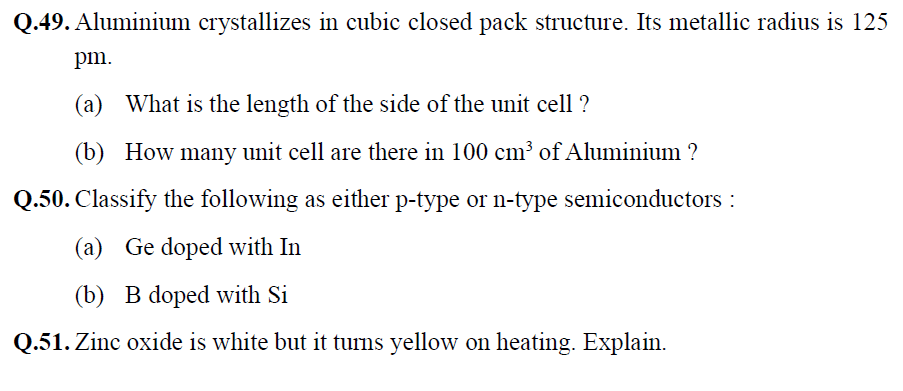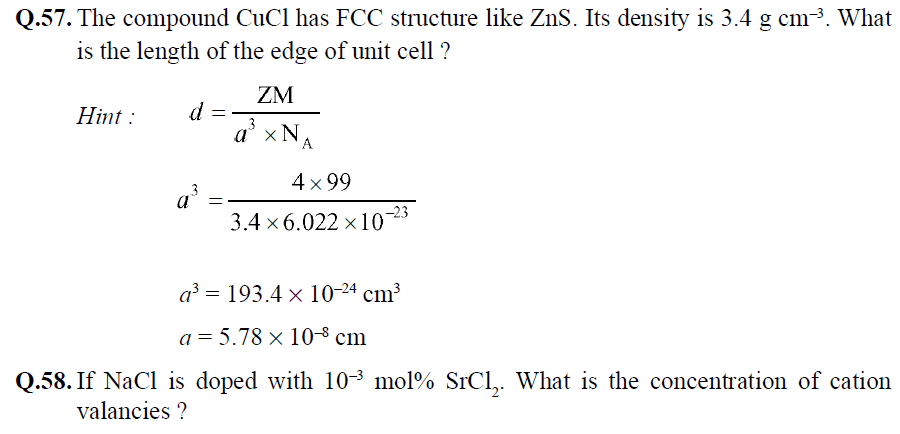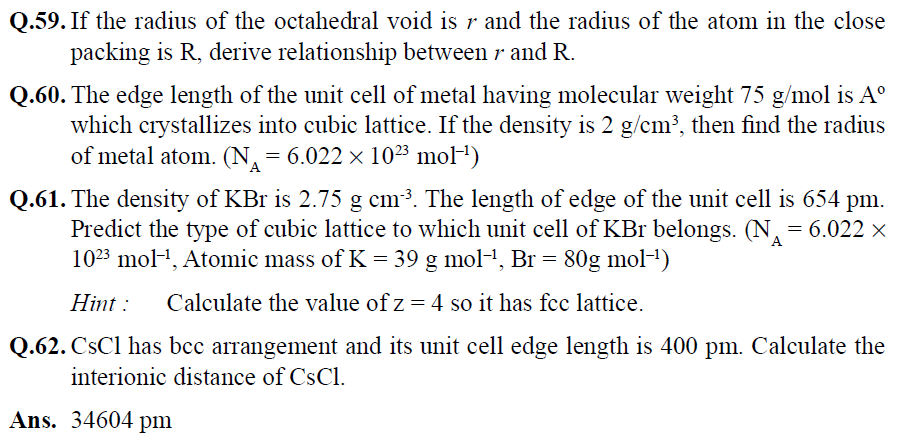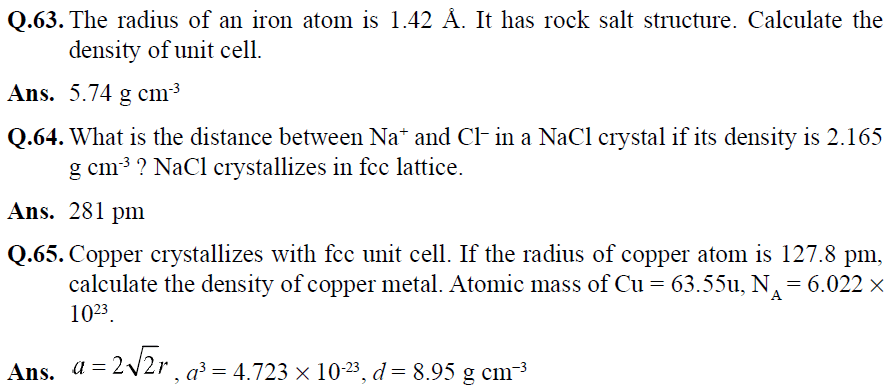CBSE Class 12 Chemistry